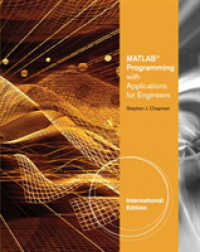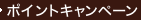•### Matlab Programming with Applications for Engineers, International Edition -- Paperback / softback （Internatio）

• ただいまウェブストアではご注文を受け付けておりません。 ⇒古書を探す
• 製本 Paperback:紙装版/ペーパーバック版
• 商品コード 9780495668084

### Full Description

MATLAB PROGRAMMING WITH APPLICATIONS FOR ENGINEERS seeks to simultaneously teach MATLAB as a technical programming language while introducing the student to many of the practical functions that make solving problems in MATLAB so much easier than in other languages. The book provides a complete introduction to the fundamentals of good procedural programming, developing good design habits that will serve a student well in any other language that he or she may pick up later. Programming topics and examples are used as a jumping off point for exploring the rich set of highly optimized application functions that are built directly into MATLAB.

### Contents

1. INTRODUCTION TO MATLAB.The Advantages of MATLAB. Disadvantages of MATLAB. The MATLAB Environment. Using MATLAB as a Calculator.2. MATLAB BASICS.Variables and Arrays. Creating and Initializing Variables in MATLAB. Multidimensional Arrays. Subarrays. Special Values. Displaying Output Data. Data Files. Scalar and Array Operations. Hierarchy of Operations. Built-in MATLAB Functions. Introduction to Plotting. Examples. MATLAB Applications: Vector Mathematics. MATLAB Applications: Matrix Operations and Simultaneous Equations. Debugging MATLAB Programs.3. TWO-DIMENSIONAL PLOTS.Additional Plotting Features for Two-Dimensional Plots. Polar Plots. Annotating and Saving Plots. Additional Types of Two-Dimensional Plots. Using the plot function with Two-Dimensional Arrays.4. BRANCHING STATEMENTS AND PROGRAM DESIGN.Introduction to Top-Down Design Techniques. Use of Pseudocode. Relational and Logic Operators. Branches. More on Debugging MATLAB Programs. MATLAB Applications: Roots of Polynomials.5. LOOPS AND VECTORIZATION.The while Loop. The for Loop. Logical Arrays and Vectorization. The MATLAB Profiler. Additional Examples. The textread Function. MATLAB Applications: Statistical Functions. MATLAB Applications: Curve Fitting and Interpolation.6. BASIC USER-DEFINED FUNCTIONS.Introduction to MATLAB Functions. Variable Passing in MATLAB: The Pass-By-Value Scheme. Optional Arguments. Sharing Data Using Global Memory. Preserving Data Between Calls to a Function. MATLAB Applications: Sorting Functions. MATLAB Applications: Random Number Functions.Function Functions. Subfunctions and Private Functions. Function Handles. Anonymous Functions. Recursive Functions. Plotting Functions. Histograms.8. COMPLEX NUMBERS AND 3D PLOTS.Complex Data. Multidimensional Arrays. Three-Dimensional Plots.9. CELL ARRAYS, STRUCTURES, AND IMPORTING DATA.Cell Arrays. Structure Arrays. Importing Data into MATLAB.10. HANDLE GRAPHICS AND ANIMATION.Handle Graphics. Position and Units. Printer Positions. Default and Factory Properties. Graphics Object Properties. Animations and Movies.11 MORE MATLAB APPLICATIONS.Solving Systems of Simultaneous Equations. Differences and Numerical Differentiation. Numerical Integration--Finding the Area Under a Curve. Differential Equations.APPENDIX A. ASCII CHARACTER SET.APPENDIX B. ADDITIONAL MATLAB INPUT / OUTPUT FUNCTIONS.MATLAB File Processing. File Opening and Closing. Binary I/O Functions. Formatted I/O Functions. The textscan Function.APPENDIX C. WORKING WITH CHARACTER STRINGS.String Functions.